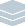Wolfram Archive

# Mathematical Typesetting(TEX, MathType, MathML, Scientific Word, troff, ...)
Ever since Mathematica 3 was released in 1996, one of the great unequalled achievements of Mathematica has been the deep integration of mathematical typesetting with computation. Mathematica lets you enter mathematical notation using the keyboard or palettes, and not only display it immediately, but also evaluate it with full mathematical meaning.
While many typesetting systems support the basic characters and layout of mathematics, Mathematica is unique in maintaining a true semantic representation. Coupled with many original algorithms, this gives Mathematica the ability to robustly typeset arbitrarily large formulas in real time, continuously linebreaking them in a semantically appropriate way. It also gives Mathematica the ability to convert between different notations and different display, presentation and input formats.
Mathematica integrates mathematical typesetting throughout the system, allowing it not only to be mixed arbitrarily with text, but also to appear in tables, graphics, menus, buttons, input fields, tooltips and anywhere else. Mathematica's mathematical typesetting is also uniquely extensible. With its unified symbolic architecture, Mathematica can represent typesetting constructs as symbolic structures that can immediately be manipulated using the full power of the Mathematica language. This makes it easy to scale from manual entry of small formulas, to the creation of simple templates and palettes, to full programmatic construction of arbitrary notation—taking mathematical typesetting to a new level of automation and sophistication.
Mathematical Typesetting System Features in Mathematica:
Key Advantages of Mathematica for Mathematical Typesetting:
• Full semantic representation of typeset expressions
• Efficient robust handling of arbitrarily large formulas
• Automatic semantically appropriate breaking of formulas into lines
• Typeset expressions can be used as input in all computations »
• Computational output generated automatically in fully editable typeset form
• Custom-designed mathematical fonts, optimized for clarity
• Antialiasing for smoother screen display
• Arbitrary coloring and styling of all parts of mathematical expressions
• Complete integration into Mathematica notebook documents
• Full integration of mathematical typesetting with table layout
• Full typesetting allowed in buttons, menus, input fields, tooltips, etc.
• Mathematical typesetting can be used inside graphics
• Graphics, tables, and arbitrary text can appear with mathematical expressions
• Support for arbitrary graphical notation
• Automatic conversion between alternative notations
• Control of typesetting styles through stylesheets
• Symbolic representation of arbitrary typeset structures
• Notation arbitrarily extensible using the full Mathematica language
• Careful mnemonic design of keyboard shortcuts for special characters and layout
• Free-form backspacing at all stages in expression entry
• Semantically aware syntax coloring for 1D and 2D expression entry
• Systemwide use of Unicode for mathematical and international characters
Interoperability with Mathematical Typesetting Systems:
• Support for both export and import of TEX
• Import and export of MathML, including semantic markup
• Immediate recognition and interpretation of TEX, MathML, etc. on the clipboard
• Import and export of standard formats including PDF, JPEG, PNG, SVG, TIFF, ...
• Import and export of RTF and other standard word-processing formats
Interesting Tidbits:
• The Wolfram Functions Site, typeset with Mathematica, is the single largest collection of formulas on the web
• Every formula in MathWorld is formatted using Mathematica
• The mathematical typesetting in all U.S. patents is done with Mathematica
• Mathematica's mathematical fonts were created by a collaboration between Wolfram Research's mathematicians and font designers
• Wolfram Research was a major force behind the MathML standard